# Solving a System of Equations with Matrices: 2 Variables

This post will provide examples of solving a system of equations with 2 variables. The primary objective of using a matrix is to perform enough row operations until you achieve what is called row-echelon form. Row-echelon form is simply having ones all across the diagonal from the top left to the bottomright with zeros underneath the dia. Below is a picture of what this looks like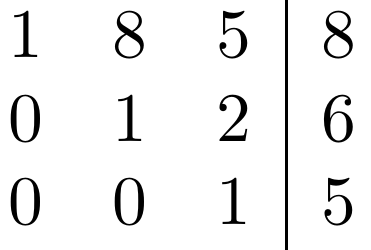It is not necessary to have ones in the diagonal it simply preferred when possible. However, you must have the zeros underneath the diagonal in order to solve the system. Every zero represents a variable that was eliminated which helps in solving for the other variables.

Two-Variable System of Equations

Our first system is as follows

# 3x + 4y = 5 x + 2y = 1

Here is our system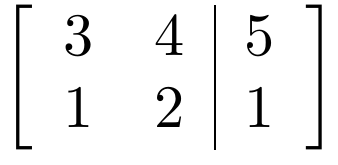Generally, for a 2X3 matrix, you start in the top left corner with the goal of converting this number into a 1.Then move to the second row of the first column and try to make this number a 0. Next, you move to the second column second row and try to make this a 1.

With this knowledge, the first-row operation we will do is flip the 2nd and 1st row. Doing this will give us a 1 in the upper left spot.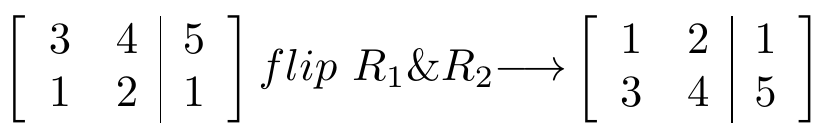Now we want in the bottom left column where the 3 is currently at. To do this we need to multiply row 1 by -3 and then add row 1 to row 2. This will give us a 0.We now need to deal with the middle row, bottom number, which is -2. To change this into a 1 we need to multiple rows to by the reciprocal of this which is -1/2.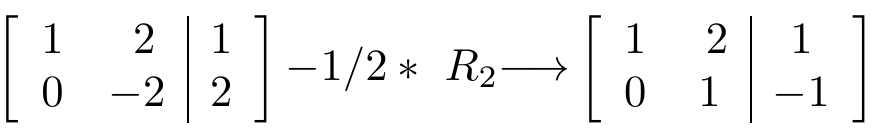If you look closely you will see that we have achieved row-echelon form. We have all 1s in the diagonal and only 0s under the diagonal.

Our new system of equations looks like the following

# 1x + 2y = 10x +1y = -1 or y = -1

If we substitute -1 for y in our top equation we can solove for x.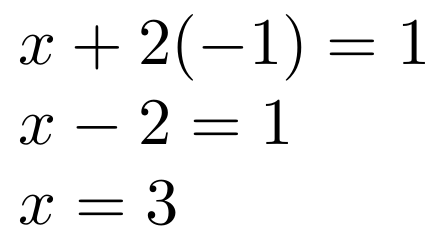We now know that x = 3 and y = -1. This indicates that we have solved our system of equations using matrices and row operations.

Conclusion

Using matrices to solve a system of equations can be cumbersome. However, once this is mastered it can often be faster than other means. In addition, understanding matrices is critical to being able to appreciate complex machine learning algorithms that almost exclusively use matrices.